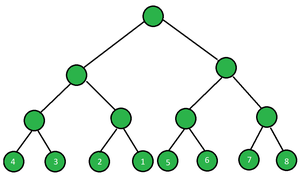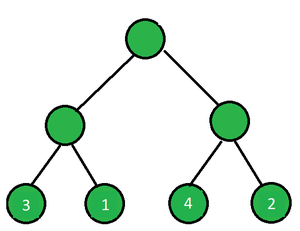Open in App
Not now

# Minimum swaps to sort the leaf nodes in a Perfect Binary Tree

• Difficulty Level : Hard
• Last Updated : 09 Dec, 2022

Given a perfect binary tree of height N  and an array of length 2N which represents the values of leaf nodes from left to right. An operation is defined as choosing any non-leaf vertex of the tree and swapping its left and right sons (along with their subtrees). Find the minimum number of such operations required to sort the value of leaf nodes in increasing order.

Examples:

Input: N = 3, leaf[] = {4, 3, 2, 1, 5, 6, 7, 8}Tree representing given input

Output: 3
Explanation: Swap the left and right part of subtree having leaf nodes 4 and 3, swap left and right part of subtree having leaf nodes 2 and 1 and finally swap left and right part of whole left subtree of root having leaf nodes as 4, 3, 2, 1. Total swap operations performed is equal to three which is minimum to sort the leaf nodes in increasing order.

Input: N=2, leaf[] = {3, 1, 4, 2}Tree representing given input

Output: -1
Explanation: We can perform two swapping operations on left and right part of subtrees having leaf nodes as 3&1 and 4&2, but we will get the leaf nodes in order {1, 3, 2, 4} which isn’t sorted. Since the leaf nodes are not sorted in increasing order so we return -1.

Approach: To solve the problem follow the below idea:

The idea/intuition is to use the divide and conquer technique, we recursively call our function for the left and right half of the tree to give the operations required to sort the leaf nodes in the left and right subtree. At last, we check if the array is sorted so we return the minimum operations otherwise if the array isn’t sorted we return -1.

Follow the steps to solve the problem:

• Initialize two pointers low = 0 and high = n – 1 and make the initial call to calculate swaps for sorting leaf nodes and result = 0.
• Find the mid pointer, mid = low + (high – low) / 2.
• Call minSwapsFunction recursively for the left subtree containing leaf nodes from 0 to mid and the right subtree containing leaf nodes from mid + 1 to high.
• Merge the left and right subtree of the non-leaf vertex, check and perform the swap if the leaf nodes in the right part are lesser than the leaf nodes in the left part, and increment the result as you swap.
• In the end, check if the leaf nodes are sorted or not, and return the result if they are sorted otherwise return -1.

## C++

```// C++ code to implement the approach

#include <bits/stdc++.h>
using namespace std;

// Function to perform swap in the left
// and right parts if required
bool mergeSubTrees(vector<int>& a, int low, int mid,
int high)
{
// If the leaf nodes are already sorted
if (a[mid] < a[mid + 1])
return 0;

// Swapping the left leaf nodes
// with right leafnodes
for (int i = low; i <= mid; i++)
swap(a[i], a[i + (high - low + 1) / 2]);
return 1;
}

// Recursive function to count the
// operations required in left and right
// subtree to make leaf nodes sorted
void minSwapsRequired(vector<int>& a, int low, int high,
int& result)
{
if (low < high) {

// Calculating middle point for
// partitioning the tree into
// left and right subtrees
int mid = low + (high - low) / 2;

// Recursive call for left subtree
minSwapsRequired(a, low, mid, result);

// Recursive call for right subtree
minSwapsRequired(a, mid + 1, high, result);

// If swap was made in left
// and right part of subtrees
// then increase the result
if (mergeSubTrees(a, low, mid, high))
result++;
}
}

// Driver code
int main()
{
int N = 3;

// Perfect tree with N height
// has 2^N leaf nodes
N = (1 << N);
vector<int> a = { 4, 3, 2, 1, 5, 6, 7, 8 };

int result = 0;

minSwapsRequired(a, 0, N - 1, result);

// Check if the leaf nodes are
// now in increasing order or not
if (is_sorted(a.begin(), a.end()))
cout << result;
else
cout << -1;

return 0;
}```

## Java

```/*package whatever //do not write package name here */

import java.io.*;
import java.util.*;

class GFG {

public static ArrayList<Integer> a
= new ArrayList<Integer>();
public static int result = 0;

public static boolean is_sorted(ArrayList<Integer> a)
{
for (int i = 1; i < a.size(); i++) {
if (a.get(i) < a.get(i - 1))
return false;
}

return true;
}

// Function to perform swap in the left
// and right parts if required
public static boolean
mergeSubTrees(ArrayList<Integer> a, int low, int mid,
int high)
{
// If the leaf nodes are already sorted
if (a.get(mid) < a.get(mid + 1)) {
return false;
}

// Swapping the left leaf nodes
// with right leafnodes
for (int i = low; i <= mid; i++) {
int tempswap = a.get(i);
a.set(i, a.get(i + (high - low + 1) / 2));
a.set(i + (high - low + 1) / 2, tempswap);
}
return true;
}

// Recursive function to count the
// operations required in left and right
// subtree to make leaf nodes sorted
public static void
minSwapsRequired(ArrayList<Integer> a, int low,
int high)
{
if (low < high) {

// Calculating middle point for
// partitioning the tree into
// left and right subtrees
int mid = low + (high - low) / 2;

// Recursive call for left subtree
minSwapsRequired(a, low, mid);

// Recursive call for right subtree
minSwapsRequired(a, mid + 1, high);

// If swap was made in left
// and right part of subtrees
// then increase the result
if (mergeSubTrees(a, low, mid, high) == true) {
result = result + 1;
}
}
}

public static void main(String[] args)
{
int N = 3;

// Perfect tree with N height
// has 2^N leaf nodes
N = (1 << N);

minSwapsRequired(a, 0, N - 1);

// Check if the leaf nodes are
// now in increasing order or not
if (is_sorted(a))
System.out.println(result);
else
System.out.println("-1");
}
}

// This code is contributed by akashish__```

## Python3

```# Python code for the above approach
result = 0;

# Function to perform swap in the left
# and right parts if required
def mergeSubTrees(a, low, mid, high):

# If the leaf nodes are already sorted
if (a[mid] < a[mid + 1]):
return 0;

# Swapping the left leaf nodes
# with right leafnodes
for i in range(low, mid + 1):
temp = a[i];
a[i] = a[i + (high - low + 1) // 2];
a[i + (high - low + 1) // 2] = temp;

return 1;

# Recursive function to count the
# operations required in left and right
# subtree to make leaf nodes sorted
def minSwapsRequired(a, low, high):
global result;
if (low < high):

# Calculating middle point for
# partitioning the tree into
# left and right subtrees
mid = (high + low) // 2;

# Recursive call for left subtree
minSwapsRequired(a, low, mid);

# Recursive call for right subtree
minSwapsRequired(a, mid + 1, high);

# If swap was made in left
# and right part of subtrees
# then increase the result
if (mergeSubTrees(a, low, mid, high)):
result += 1

def is_sorted(a):
for i in range(1, len(a)):
if (a[i] < a[i - 1]):
return 0;

return 1;

# Driver code
N = 3;

# Perfect tree with N height
# has 2^N leaf nodes
N = (1 << N);
a = [4, 3, 2, 1, 5, 6, 7, 8];

minSwapsRequired(a, 0, N - 1);

# Check if the leaf nodes are
# now in increasing order or not
if (is_sorted(a)):
print(result);
else:
print(-1);

# This code is contributed by Saurabh Jaiswal
```

## C#

```// C# code to implement the approach
using System;
public class GFG {

public static bool is_sorted(int[] a)
{
for (int i = 1; i < a.Length; i++) {
if (a[i] < a[i - 1])
return false;
}

return true;
}

static void swap(ref int x, ref int y)
{
int tempswap = x;
x = y;
y = tempswap;
}

// Function to perform swap in the left
// and right parts if required
public static bool mergeSubTrees(ref int[] a, int low,
int mid, int high)
{
// If the leaf nodes are already sorted
if (a[mid] < a[mid + 1])
return false;

// Swapping the left leaf nodes
// with right leafnodes
for (int i = low; i <= mid; i++)
swap(ref a[i], ref a[i + (high - low + 1) / 2]);
return true;
}

// Recursive function to count the
// operations required in left and right
// subtree to make leaf nodes sorted
public static void minSwapsRequired(ref int[] a,
int low, int high,
ref int result)
{
if (low < high) {

// Calculating middle point for
// partitioning the tree into
// left and right subtrees
int mid = low + (high - low) / 2;

// Recursive call for left subtree
minSwapsRequired(ref a, low, mid, ref result);

// Recursive call for right subtree
minSwapsRequired(ref a, mid + 1, high,
ref result);

// If swap was made in left
// and right part of subtrees
// then increase the result
if (mergeSubTrees(ref a, low, mid, high))
result++;
}
}

// Driver code
static public void Main()
{

int N = 3;

// Perfect tree with N height
// has 2^N leaf nodes
N = (1 << N);
int[] a = { 4, 3, 2, 1, 5, 6, 7, 8 };

int result = 0;

minSwapsRequired(ref a, 0, N - 1, ref result);

// Check if the leaf nodes are
// now in increasing order or not
if (is_sorted(a))
Console.WriteLine(result);
else
Console.WriteLine(-1);
}
}

// This code is contributed by akashish__```

## Javascript

```<script>
// JavaScript code for the above approach
var result = 0;

// Function to perform swap in the left
// and right parts if required
function mergeSubTrees(a, low, mid,
high)
{

// If the leaf nodes are already sorted
if (a[mid] < a[mid + 1])
return 0;

// Swapping the left leaf nodes
// with right leafnodes
for (let i = low; i <= mid; i++) {
let temp = a[i];
a[i] = a[i + Math.floor((high - low + 1) / 2)];
a[i + Math.floor((high - low + 1) / 2)] = temp;
}
return 1;
}

// Recursive function to count the
// operations required in left and right
// subtree to make leaf nodes sorted
function minSwapsRequired(a, low, high
) {
if (low < high) {

// Calculating middle point for
// partitioning the tree into
// left and right subtrees
let mid = Math.floor((high + low) / 2);

// Recursive call for left subtree
minSwapsRequired(a, low, mid);

// Recursive call for right subtree
minSwapsRequired(a, mid + 1, high);

// If swap was made in left
// and right part of subtrees
// then increase the result
if (mergeSubTrees(a, low, mid, high))
result++;
}
}

function is_sorted(a) {
for (let i = 1; i < a.length; i++) {
if (a[i] < a[i - 1])
return 0;
}

return 1;
}

// Driver code
let N = 3;

// Perfect tree with N height
// has 2^N leaf nodes
N = (1 << N);
var a = [4, 3, 2, 1, 5, 6, 7, 8];

minSwapsRequired(a, 0, N - 1, result);

// Check if the leaf nodes are
// now in increasing order or not
if (is_sorted(a))
document.write(result);
else
document.write(-1);

// This code is contributed by Potta Lokesh

</script>```
Output

`3`

Time Complexity: O(N * log N)
Auxiliary Space: O(N), recursion stack space

My Personal Notes arrow_drop_up
Related Articles